# Power Analysis

## Overview

Power analysis is an important aspect of experimental design. It allows us to determine the sample size required to detect an effect of a given size with a given degree of confidence. Conversely, it allows us to determine the probability of detecting an effect of a given size with a given level of confidence, under sample size constraints. If the probability is unacceptably low, we would be wise to alter or abandon the experiment.

The following four quantities have an intimate relationship:

1. sample size
2. effect size
3. significance level = P(Type I error) = probability of finding an effect that is not there
4. power = 1 - P(Type II error) = probability of finding an effect that is there

Given any three, we can determine the fourth.

## Power Analysis in R

The pwr package develped by Stéphane Champely, impliments power analysis as outlined by Cohen (!988). Some of the more important functions are listed below.

function power calculations for
pwr.2p.test two proportions (equal n)
pwr.2p2n.test two proportions (unequal n)
pwr.anova.test balanced one way ANOVA
pwr.chisq.test chi-square test
pwr.f2.test general linear model
pwr.p.test proportion (one sample)
pwr.r.test correlation
pwr.t.test t-tests (one sample, 2 sample, paired)
pwr.t2n.test t-test (two samples with unequal n)

For each of these functions, you enter three of the four quantities (effect size, sample size, significance level, power) and the fourth is calculated.

The significance level defaults to 0.05. Therefore, to calculate the significance level, given an effect size, sample size, and power, use the option "sig.level=NULL".

Specifying an effect size can be a daunting task. ES formulas and Cohen's suggestions (based on social science research) are provided below. Cohen's suggestions should only be seen as very rough guidelines. Your own subject matter experience should be brought to bear.

(To explore confidence intervals and drawing conclusions from samples try this interactive course on the foundations of inference.)

## t-tests

For t-tests, use the following functions:

pwr.t.test(n = , d = , sig.level = , power = , type = c("two.sample", "one.sample", "paired"))

where n is the sample size, d is the effect size, and type indicates a two-sample t-test, one-sample t-test or paired t-test. If you have unequal sample sizes, use

pwr.t2n.test(n1 = , n2= , d = , sig.level =, power = )

where n1 and n2 are the sample sizes.

For t-tests, the effect size is assessed as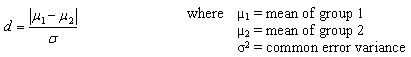Cohen suggests that d values of 0.2, 0.5, and 0.8 represent small, medium, and large effect sizes respectively.

You can specify alternative="two.sided", "less", or "greater" to indicate a two-tailed, or one-tailed test. A two tailed test is the default.

## ANOVA

For a one-way analysis of variance use

pwr.anova.test(k = , n = , f = , sig.level = , power = )

where k is the number of groups and n is the common sample size in each group.

For a one-way ANOVA effect size is measured by f where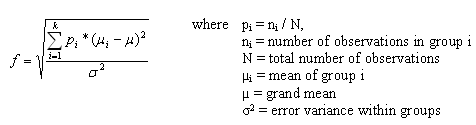Cohen suggests that f values of 0.1, 0.25, and 0.4 represent small, medium, and large effect sizes respectively.

## Correlations

For correlation coefficients use

pwr.r.test(n = , r = , sig.level = , power = )

where n is the sample size and r is the correlation. We use the population correlation coefficient as the effect size measure. Cohen suggests that r values of 0.1, 0.3, and 0.5 represent small, medium, and large effect sizes respectively.

## Linear Models

For linear models (e.g., multiple regression) use

pwr.f2.test(u =, v = , f2 = , sig.level = , power = )

where u and v are the numerator and denominator degrees of freedom. We use f2 as the effect size measure.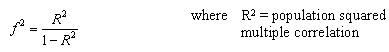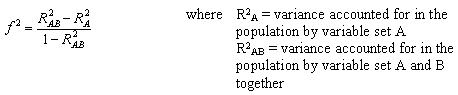The first formula is appropriate when we are evaluating the impact of a set of predictors on an outcome. The second formula is appropriate when we are evaluating the impact of one set of predictors above and beyond a second set of predictors (or covariates). Cohen suggests f2 values of 0.02, 0.15, and 0.35 represent small, medium, and large effect sizes.

## Tests of Proportions

When comparing two proportions use

pwr.2p.test(h = , n = , sig.level =, power = )

where h is the effect size and n is the common sample size in each group.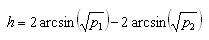Cohen suggests that h values of 0.2, 0.5, and 0.8 represent small, medium, and large effect sizes respectively.

For unequal n's use

pwr.2p2n.test(h = , n1 = , n2 = , sig.level = , power = )

To test a single proportion use

pwr.p.test(h = , n = , sig.level = power = )

For both two sample and one sample proportion tests, you can specify alternative="two.sided", "less", or "greater" to indicate a two-tailed, or one-tailed test. A two tailed test is the default.

## Chi-square Tests

For chi-square tests use

pwr.chisq.test(w =, N = , df = , sig.level =, power = )

where w is the effect size, N is the total sample size, and df is the degrees of freedom. The effect size w is defined as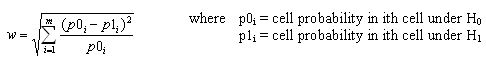Cohen suggests that w values of 0.1, 0.3, and 0.5 represent small, medium, and large effect sizes respectively.

## Some Examples

``````library(pwr)

# For a one-way ANOVA comparing 5 groups, calculate the
# sample size needed in each group to obtain a power of
# 0.80, when the effect size is moderate (0.25) and a
# significance level of 0.05 is employed.

pwr.anova.test(k=5,f=.25,sig.level=.05,power=.8)

# What is the power of a one-tailed t-test, with a
# significance level of 0.01, 25 people in each group,
# and an effect size equal to 0.75?

pwr.t.test(n=25,d=0.75,sig.level=.01,alternative="greater")

# Using a two-tailed test proportions, and assuming a
# significance level of 0.01 and a common sample size of
# 30 for each proportion, what effect size can be detected
# with a power of .75?

pwr.2p.test(n=30,sig.level=0.01,power=0.75)
``````

## Creating Power or Sample Size Plots

The functions in the pwr package can be used to generate power and sample size graphs.

``````# Plot sample size curves for detecting correlations of
# various sizes.

library(pwr)

# range of correlations
r <- seq(.1,.5,.01)
nr <- length(r)

# power values
p <- seq(.4,.9,.1)
np <- length(p)

# obtain sample sizes
samsize <- array(numeric(nr*np), dim=c(nr,np))
for (i in 1:np){
for (j in 1:nr){
result <- pwr.r.test(n = NULL, r = r[j],
sig.level = .05, power = p[i],
alternative = "two.sided")
samsize[j,i] <- ceiling(result\$n)
}
}

# set up graph
xrange <- range(r)
yrange <- round(range(samsize))
colors <- rainbow(length(p))
plot(xrange, yrange, type="n",
xlab="Correlation Coefficient (r)",
ylab="Sample Size (n)" )

for (i in 1:np){
lines(r, samsize[,i], type="l", lwd=2, col=colors[i])
}

# add annotation (grid lines, title, legend)
abline(v=0, h=seq(0,yrange,50), lty=2, col="grey89")
abline(h=0, v=seq(xrange,xrange,.02), lty=2,
col="grey89")
title("Sample Size Estimation for Correlation Studies\n
Sig=0.05 (Two-tailed)")
legend("topright", title="Power",
as.character(p),
fill=colors)
``````